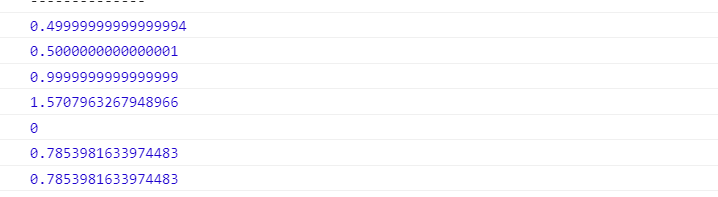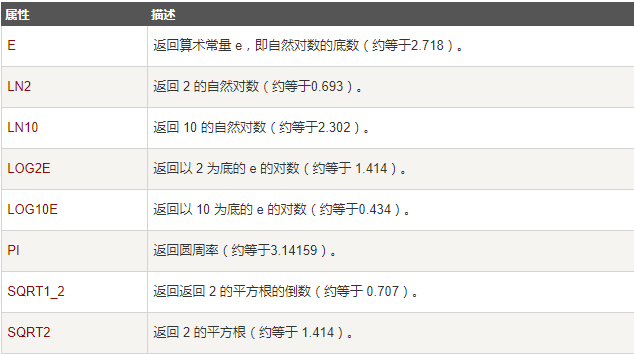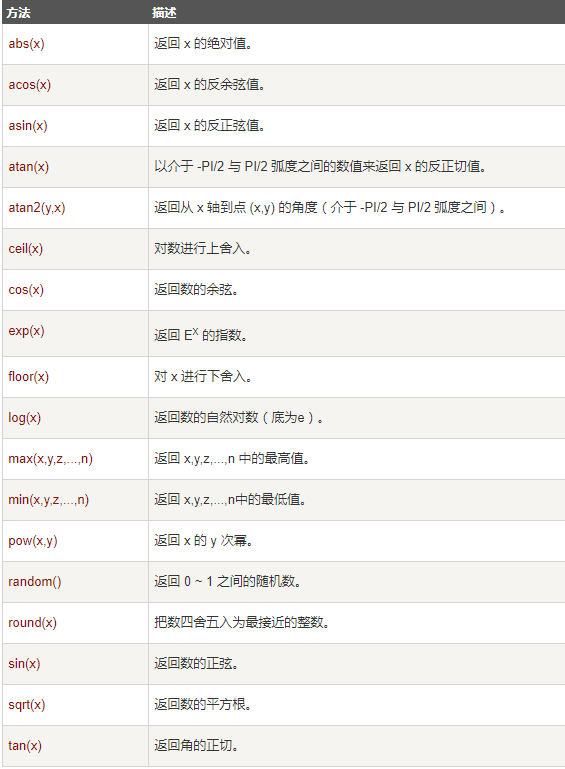• Math对象一、Math对象简介二、Math对象属性三、Math对象方法 一、Math对象简介 Math 对象用于执行数学任务。Math 对象并不像 Date 和 String 那样是对象的类，因此没有构造函数 Math()，像 Math.sin() 这样的函数...
Math对象一、Math对象简介二、Math对象属性1. E（1）属性描述（2）具体演示2. PI（1）属性描述（2）具体演示3. LN2、LN10（1）属性描述（2）具体演示4. LOG2E、LOG10E（1）属性描述（2）具体演示5. SQRT2、SQRT1_2（1）属性描述（2）具体演示三、Math对象方法1. abs()2. random()3. min()、max()4. floor()、ceil()、round()5. pow()、sqrt()、exp()、log()6. sin()、cos()、tan()、asin()、acos()、atan()、atan2()
一、Math对象简介
Math 对象用于执行数学任务。Math 对象并不像 Date 和 String 那样是对象的类，因此没有构造函数 Math()，像 Math.sin() 这样的函数只是函数，不是某个对象的方法。您无需创建它，通过把 Math 作为对象使用就可以调用其所有属性和方法。
二、Math对象属性
1. E
（1）属性描述
Math.E：返回算术常量 e，即自然对数的底数（约等于2.718）。
（2）具体演示
console.log(Math.E);

2. PI
（1）属性描述
Math.PI：返回圆周率（约等于3.14159）。
（2）具体演示
  console.log(Math.PI);

3. LN2、LN10
（1）属性描述
Math.LN2：返回 2 的自然对数（约等于0.693）。
Math.LN10：返回 10 的自然对数（约等于2.302）。
（2）具体演示
console.log(Math.LN2);
console.log(Math.LN10);

4. LOG2E、LOG10E
（1）属性描述
Math.LOG2E：返回以 2 为底的 e 的对数（约等于 1.414）。
Math.LOG10E：返回以 10为底的 e 的对数（约等于 0.434）。
（2）具体演示
console.log(Math.LOG2E);
console.log(Math.LOG10E);

5. SQRT2、SQRT1_2
（1）属性描述
Math.SQRT2：返回 2 的平方根（约等于 1.414）。
Math.SQRT1_2：返回 2 的平方根的倒数（约等于  0.707）。
（2）具体演示
console.log(Math.SQRT2);
console.log(Math.SQRT1_2);

三、Math对象方法
1. abs()
abs(x)：返回绝对值
  let a = -10
console.log(Math.abs(a)); // 10

2. random()
random()：返回 0 ~ 1 之间的随机数。
  console.log(Math.random());

3. min()、max()
min(x,y,z...)：返回最小值
max(x,y,z...)：返回最大值
   let a = 1, b = 2
console.log(Math.min(a, b)); // 1
console.log(Math.max(a, b)); // 2

4. floor()、ceil()、round()
floor(x)：向下取整
ceil(x)：向上取整
round(x)：四舍五入
let a = 10.5
console.log(Math.floor(a)); // 10
console.log(Math.ceil(a)); // 11
console.log(Math.round(a)); // 11

5. pow()、sqrt()、exp()、log()
pow(x,y)：返回x的y次方
sqrt(x)：返回数的平方根
exp(x)：返回 e 的指数
log(x)：返回数的自然对数（底为e）
    let a = Math.E
console.log(Math.pow(2, 2)); // 4
console.log(Math.sqrt(4)); // 2
console.log(Math.exp(2)); // 7.38905609893065
console.log(Math.log(a)); // 1

6. sin()、cos()、tan()、asin()、acos()、atan()、atan2()
sin(x)：正弦
cos(x)：余弦
tan(x)：正切
asin(x)：反正弦
acos(x)：反余弦
atan(x)：反正切
atan2(x,y)：x周到点x,y的角度
    let a = Math.PI / 6, b =  Math.PI / 4, c = Math.PI / 3
console.log(Math.sin(a));
console.log(Math.cos(c));
console.log(Math.tan(b));
console.log(Math.asin(1));
console.log(Math.acos(1));
console.log(Math.atan(1));
console.log(Math.atan2(1, 1));展开全文javascript
• Math 对象 Math（算数）对象的作用是：执行普通的算数任务。 Math 对象提供多种算数值类型和函数。无需在使用这个对象之前对它进行定义。 Math 对象属性 Math 对象方法 //随机myMin(包含)到myMax(包含)之间的数 ...
Math 对象

Math（算数）对象的作用是：执行普通的算数任务。
Math 对象提供多种算数值类型和函数。无需在使用这个对象之前对它进行定义。

Math 对象属性Math 对象方法//随机myMin(包含)到myMax(包含)之间的数
function randomRange(myMin,myMax){
return Math.floor(Math.random() * ( myMax -myMin + 1) ) + myMin;
}



展开全文• math对象，是提供给开发者的内置常见数学公式的对象，所有math对象中的方法都会有返回值 max( x , y ) 返回x、y中的最大值 min( x , y ) 返回x，y中的最小值 Math.random（ ） 返回0~1之间的随机数 Math abs（ a ）...
math对象，是提供给开发者的内置常见数学公式的对象，所有math对象中的方法都会有返回值

max( x , y ) 返回x、y中的最大值
min( x , y ) 返回x，y中的最小值
Math.random（ ） 返回0~1之间的随机数
Math abs（ a ）返回a的绝对值
Math. e xp ( a ) 返回e的a次方
Math.log ( a ) 返回a的自然对数(底数为e)
Math.pow( x , y ) 返回x的y次方的值
Math.sqrt （ a ）返回a的平方根
Math.random( )*a 返回0-a之间的随机数
Math.random*(较大的数 - 较小的数)+较小的数 返回两个数字之间的随机数
取整：
Math.ceil( )；  向上取整
Math.floor( )；向下取整
Math.round()；四舍五入

三原色
通过三个数字来指定颜色，通过英文名称来赋值
background-color:rgb( a , b , c)
数字的取值范围在0-256之间
opacity: ;
opacity属性：透明度，取值范围为0(全透明)~1(不透明)
background-color:rgba( a , b , c , d)
其中，a即代表opacity


展开全文...